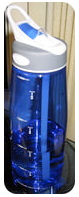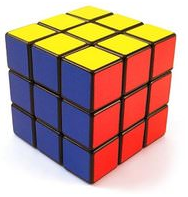3.4: SI Length and Volume Units

Introduction

Back in the days before all the electronic gadgets for measuring depth and locating undersea objects existed, the "fathom" was the unit of measurement for depth. A rope was knotted every six feet and the end was dropped over the side of the ship. You could tell how deep the water was by how many knots went under the water before the rope hit bottom. Today we just turn on an instrument and read the depth to a high level of accuracy.

Length and Volume

Length is the measurement of the extent of something along its greatest dimension. The SI basic unit of length, or linear measure, is the meter $$\left( \text{m} \right)$$. All measurements of length may be made in meters, though the prefixes listed in various tables will often be more convenient. The width of a room may be expressed as about 5 meters $$\left( \text{m} \right)$$, whereas a large distance, such as the distance between New York City and Chicago, is better expressed as 1150 kilometers $$\left( \text{km} \right)$$. Very small distances can be expressed in units such as the millimeter or the micrometer. The width of a typical human hair is about 10 micrometers $$\left( \mu \text{m} \right)$$.

Volume is the amount of space occupied by a sample of matter. The volume of a regular object can be calculated by multiplying its length by its width and its height. Since each of those is a linear measurement, we say that units of volume are derived from units of length. The SI unit of volume is the cubic meter $$\left( \text{m}^3 \right)$$, which is the volume occupied by a cube that measures $$1 \: \text{m}$$ on each side. This very large volume is not very convenient for typical use in a chemistry laboratory. A liter $$\left( \text{L} \right)$$ is the volume of a cube that measures $$10 \: \text{cm}$$ $$\left( 1 \: \text{dm} \right)$$ on each side. A liter is thus equal to both $$1000 \: \text{cm}^3$$ $$\left( 10 \: \text{cm} \times 10 \: \text{cm} \times 10 \: \text{cm} \right)$$ and to $$1 \: \text{dm}^3$$. A smaller unit of volume that is commonly used is the milliliter ($$\text{mL}$$ - note the capital $$\text{L}$$ which is a standard practice). A milliliter is the volume of a cube that measures $$1 \: \text{cm}$$ on each side. Therefore, a milliliter is equal to a cubic centimeter $$\left( \text{cm}^3 \right)$$. There are $$1000 \: \text{mL}$$ in $$1 \: \text{L}$$, which is the same as saying that there are $$1000 \: \text{cm}^3$$ in $$1 \: \text{dm}^3$$.A typical water bottle is 1 liter in volume.Rubik's cube. This Rubik's cube is $$5.7 \: \text{cm}$$ on each side and has a volume of $$185.2 \: \text{cm}^3$$ or $$185.2 \: \text{mL}$$.Graduated cylinder. Volume in the laboratory is often measured with a graduated cylinder, which comes in a variety of sizes.

Summary

• Length is the measurement of the extent of something along its greatest dimension.
• Volume is the amount of space occupied by a sample of matter.
• Volume can be determined by knowing the length of each side of the item.

Explore More

Read the section of length and volume in the link below and answer the following questions:

1. What are some units of length and volume in the metric system?

2. How long is a meter in English units?

3. How many centimeters are in an inch?

4. How many miles is a $$10 \: \text{km}$$ race?

5. What is the difference between a cubic centimeter and a milliliter?

Contributors

• CK-12 Foundation by Sharon Bewick, Richard Parsons, Therese Forsythe, Shonna Robinson, and Jean Dupon.